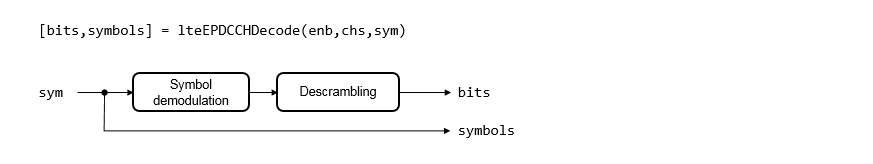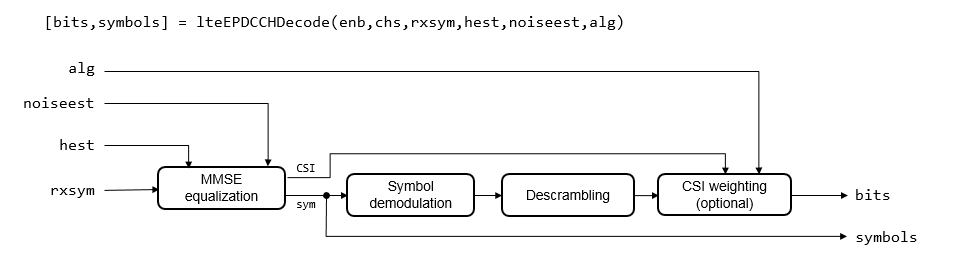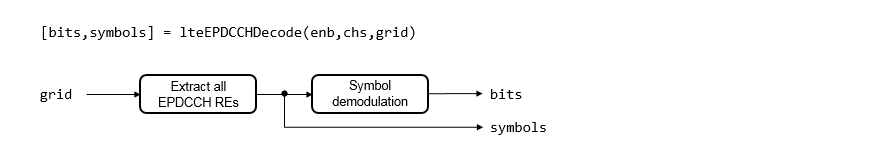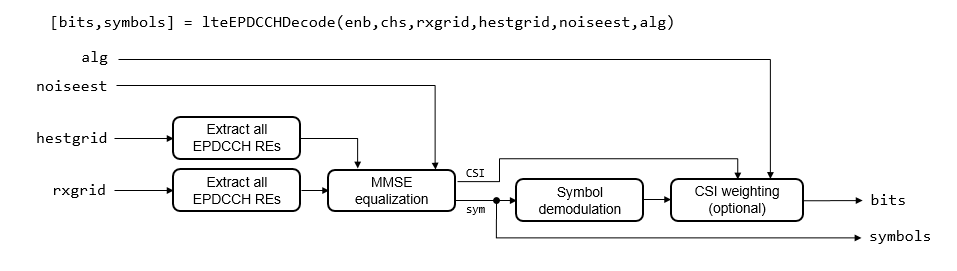Documentation

# lteEPDCCHDecode

Enhanced physical downlink control channel (EPDCCH) decoding

## Syntax

``````[bits,symbols] = lteEPDCCHDecode(enb,chs,sym)``````
``````[bits,symbols] = lteEPDCCHDecode(enb,chs,rxsym,hest,noiseest)``````
``````[bits,symbols] = lteEPDCCHDecode(enb,chs,rxsym,hest,noiseest,alg)``````
``````[bits,symbols] = lteEPDCCHDecode(enb,chs,grid)``````
``````[bits,symbols] = lteEPDCCHDecode(enb,chs,rxgrid,hestgrid,noiseest,alg)``````

## Description

example

``````[bits,symbols] = lteEPDCCHDecode(enb,chs,sym)``` returns softbits and received constellation of complex symbols resulting from performing the inverse of enhanced physical downlink control channel (EPDCCH) processing of a single configured EPDCCH candidate given cell-wide settings structure, EPDCCH transmission configuration structure, and EPDCCH symbols. The input symbols are assumed to contain ideal EPDCCH symbols, so no equalization is performed. The output received EPDCCH symbols are QPSK symbol demodulated and descrambled. For more EPDCCH processing information, see `lteEPDCCH` and TS 36.211, Section 6.8A. When using this syntax, the input structures only require `enb`.`NSubframe` and `chs`.`EPDCCHNID`. For more information, see Syntax Dependent Processing.```

example

``````[bits,symbols] = lteEPDCCHDecode(enb,chs,rxsym,hest,noiseest)``` performs EPDCCH decoding and equalization for a single configured EPDCCH candidate given cell-wide settings structure, EPDCCH transmission configuration structure, received EPDCCH symbols `rxsym`, channel estimate `hest`, and noise estimate `noiseest`. The output received EPDCCH symbols are equalized, and QPSK symbol demodulated and descrambled. ```
``````[bits,symbols] = lteEPDCCHDecode(enb,chs,rxsym,hest,noiseest,alg)``` performs EPDCCH decoding and equalization for a single configured EPDCCH candidate and provides control over weighting the output soft bits with channel state information (CSI) calculated during the equalization stage using algorithmic configuration structure, `alg`.```
``````[bits,symbols] = lteEPDCCHDecode(enb,chs,grid)``` performs EPDCCH decoding for all possible EPDCCH candidate locations given cell-wide settings structure, EPDCCH transmission configuration structure, and the resource element grid across all possible EPDCCH antenna ports. The resource element grid is assumed to contain ideal EPDCCH REs, so no equalization is performed. The decoding consists of extraction of all EPDCCH REs from `grid` followed by QPSK symbol demodulation. Each EPDCCH candidate is descrambled individually during EPDCCH search. For this syntax, `chs`.`EPDCCHECCE` and `chs`.`EPDCCHNID` are not required as no candidate-specific resource extraction or descrambling is performed.```
``````[bits,symbols] = lteEPDCCHDecode(enb,chs,rxgrid,hestgrid,noiseest,alg)``` performs EPDCCH decoding and equalization for all possible EPDCCH candidate locations given cell-wide settings structure, EPDCCH transmission configuration structure, received resource element grid, channel estimate grid, noise estimate, and provides control over weighting the output soft bits with channel state information (CSI) calculated during the equalization stage using algorithmic configuration structure, `alg`. EPDCCH RE locations extracted from `rxgrid` and `hestgrid` are equalized, then QPSK symbol demodulated. Each EPDCCH candidate is descrambled individually during EPDCCH search. For this syntax, `chs`.`EPDCCHECCE` and `chs`.`EPDCCHNID` are not required as no candidate-specific resource extraction or descrambling is performed. ```

## Examples

collapse all

Modulate and then demodulate EPDCCH symbols for a codeword of random bits.

Initialize the cell-wide settings structure and the EPDCCH transmission channel configuration structure.

```enb.NSubframe = 0; chs.EPDCCHNID = 1;```

Create an input codeword for EPDCCH and generate EPDCCH symbols.

```cw = randi([0 1],108,1); sym = lteEPDCCH(enb,chs,cw);```

Decode the symbols and confirm the codeword was successfully recovered.

```rxcw = lteEPDCCHDecode(enb,chs,sym); isequal(cw,rxcw>0)```
```ans = logical 1 ```

Perform DCI coding to the capacity of a particular EPDCCH candidate. EPDCCH modulate the coded message and transmit it. Add EPDCCH demodulation reference signals (DMRS) and perform channel estimation. Finally, extract the EPDCCH (and corresponding channel estimate) from the resource grid. Perform EPDCCH demodulation and decode the received DCI message.

Initialize the cell-wide settings structure.

```enb.NSubframe = 0; enb.NDLRB = 15; enb.CyclicPrefix = 'Extended'; enb.CellRefP = 2; enb.NCellID = 1; enb.CFI = 1;```

Initialize the EPDCCH transmission channel configuration structure.

```chs.EPDCCHNID = 1; chs.EPDCCHPRBSet = (0:3).'; chs.EPDCCHType = 'Localized'; chs.EPDCCHFormat = 2; chs.ControlChannelType = 'EPDCCH'; chs.DCIFormat = 'Format2D'; chs.RNTI = 11;```

Create a set of random bits representing a DCI message and performing DCI coding to the capacity of a particular EPDCCH candidate.

```dciInfo = lteDCIInfo(enb,chs); dcibits = randi([0 1],dciInfo.(chs.DCIFormat),1); candidates = lteEPDCCHSpace(enb,chs); chs.EPDCCHECCE = candidates(1,:); [ind,info] = lteEPDCCHIndices(enb,chs); cw = lteDCIEncode(chs,dcibits,info.EPDCCHG);```

Generate EPDCCH symbols and resource element grid. Populate the grid.

```sym = lteEPDCCH(enb,chs,cw); grid = lteDLResourceGrid(enb,4); grid(ind) = sym; grid(lteEPDCCHDMRSIndices(enb,chs)) = lteEPDCCHDMRS(enb,chs);```

Generate channel estimate.

```cec.PilotAverage = 'TestEVM'; cec.Reference = 'EPDCCHDMRS'; [hestgrid,noiseest] = lteDLChannelEstimate(enb,chs,cec,grid); [rxsym,hest] = lteExtractResources(ind,grid,hestgrid);```

Decode the symbols and DCI message bits. Confirm the DCI message was successfully recovered.

```rxcw = lteEPDCCHDecode(enb,chs,rxsym,hest,noiseest); rxdcibits = lteDCIDecode(dciInfo.(chs.DCIFormat),rxcw); isequal(dcibits,rxdcibits>0)```
```ans = logical 1 ```

## Input Arguments

collapse all

eNodeB cell-wide settings, specified as a structure containing these parameter fields.

Parameter FieldRequired or OptionalValuesDescription
`NDLRB`Required

Scalar integer from 6 to 110

Number of downlink resource blocks. (${N}_{\text{RB}}^{\text{DL}}$)

`NCellID`Required

Integer from 0 to 503

Physical layer cell identity

`CyclicPrefix`Optional

`'Normal'` (default), `'Extended'`

Cyclic prefix length

`CellRefP`Required

1, 2, 4

Number of cell-specific reference signal (CRS) antenna ports

`NSubframe`Required

0 (default), nonnegative scalar integer

Subframe number

The following parameter is only read when `chs`.`EPDCCHStart` is not present.

`CFI`Required

1, 2, or 3
Scalar or if the CFI varies per subframe, a vector of length 10 (corresponding to a frame).

Control format indicator (`CFI`) value. In TDD mode, `CFI` varies per subframe for the RMCs (`'R.0', 'R.5', 'R.6', 'R.6-27RB', 'R.12-9RB'`)

`DuplexMode`Optional

`'FDD'` (default), `'TDD'`

Duplexing mode, specified as one of the following:

• `'FDD'` — Frequency division duplex (default)

• `'TDD'` — Time division duplex

The following parameters apply when `DuplexMode` is set to `'TDD'`.

`TDDConfig`Optional

0, 1 (default), 2, 3, 4, 5, 6

`SSC`Optional

0 (default), 1, 2, 3, 4, 5, 6, 7, 8, 9

Special subframe configuration (SSC)

`NFrame`Optional

0 (default), nonnegative scalar integer

Frame number

`CSIRSPeriod`Optional

`'Off'` (default), `'On'`, `Icsi-rs` (0,...,154), ```[Tcsi-rs Dcsi-rs]```. You can also specify values in a cell array of configurations for each resource.

CSI-RS subframe configurations for one or more CSI-RS resources. Multiple CSI-RS resources can be configured from a single common subframe configuration or from a cell array of configurations for each resource.

The following CSI-RS resource parameters apply only when `CSIRSPeriod` sets one or more CSI-RS subframe configurations to any value other than `'Off'`. Each parameter length must be equal to the number of CSI-RS resources required.

`CSIRSConfig`Required

Nonnegative scalar integer

Array CSI-RS configuration indices. See TS 36.211, Table 6.10.5.2-1.

`CSIRefP`Required

1 (default), 2, 4, 8

Array of number of CSI-RS antenna ports

`ZeroPowerCSIRSPeriod`Optional

`'Off'` (default), `'On'`, `Icsi-rs` (0,...,154), ```[Tcsi-rs Dcsi-rs]```. You can also specify values in a cell array of configurations for each resource.

Zero power CSI-RS subframe configurations for one or more zero power CSI-RS resource configuration index lists. Multiple zero power CSI-RS resource lists can be configured from a single common subframe configuration or from a cell array of configurations for each resource list.

The following zero power CSI-RS resource parameter is only applicable if one or more of the above zero power subframe configurations are set to any value other than `'Off'`.

`ZeroPowerCSIRSConfig`Required

16-bit bitmap character vector or string scalar (truncated if not 16 bits or `'0'` MSB extended), or a numeric list of CSI-RS configuration indices. You can also specify values in a cell array of configurations for each resource.

Zero power CSI-RS resource configuration index lists (TS 36.211 Section 6.10.5.2). Specify each list as a 16-bit bitmap character vector or string scalar (if less than 16 bits, then `'0'` MSB extended), or as a numeric list of CSI-RS configuration indices from TS 36.211 Table 6.10.5.2-1 in the `'4'` CSI reference signal column. Multiple lists can be defined using a cell array of individual lists.

EPDCCH-specific channel transmission configuration, specified as a structure that can contain the following parameter fields.

Parameter FieldRequired or OptionalValuesDescription
`EPDCCHECCE`Required

1- or 2- element vector specifying the zero-based ECCE index or inclusive [begin, end] ECCE index range according to the aggregation level `L`, where L = end – begin + 1. The number of ECCEs in the candidate must be a power of 2.

If no transmission is required, leave this parameter empty.

The set of one of several consecutive ECCEs defining the EPDCCH transmission candidate in the overall EPDCCH set.

`EPDCCHType`Required

`'Localized'`, `'Distributed'`

EPDCCH transmission type

`EPDCCHPRBSet`Required

Vector of zero-based indices for the PRB pairs corresponding to the EPDCCH PRB set. The number of PRB pair indices must be a power of 2.

If no transmission is required, leave this parameter empty.

EPDCCH PRB pair indices

`EPDCCHStart`Optional

integer from 0 to 4

If this parameter is not present, then the cell-wide CFI parameter is used for the starting symbol.

EPDCCH starting symbol

`EPDCCHNID`Required

nonnegative scalar integer

EPDCCH scrambling sequence initialization

The following parameter applies when `EPDCCHType` is set to `'Localized'`.

`RNTI`Required

0 (default), scalar integer

Radio network temporary identifier (RNTI) value (16 bits)

`EPDCCH` modulation symbols associated with a single `EPDCCH` transmission in a subframe, specified as a complex vector. This input contains the QPSK symbols.

Data Types: `double`

Received EPDCCH symbols, specified as a EPDCCHGd-by-NRxAnts matrix. EPDCCHGd is the EPDCCH symbol capacity, given by the `info.EPDCCHGd` field of `lteEPDCCHIndices`. NRxAnts is the number of receive antennas. This matrix contains the elements of the received resource grid in the locations of the EPDCCH REs for the candidate configured via `chs`.`EPDCCHECCE`.

Data Types: `double`

Channel estimate, specified as an EPDCCHGd-by-NRxAnts array. EPDCCHGd is the EPDCCH symbol capacity, given by the `info.EPDCCHGd` field of `lteEPDCCHIndices`. NRxAnts is the number of receive antennas. The third dimension represents the 4 possible EPDCCH antenna ports (p=107...110). This array contains the elements of the channel estimate array in the locations of the EPDCCH REs for the candidate configured via `chs`.`EPDCCHECCE`.

Data Types: `double`

Noise estimate of the noise power spectral density per RE on the received subframe, specified as a numeric scalar. The ` lteDLChannelEstimate` function provides this estimate.

Data Types: `double`

Algorithmic configuration, specified as a structure. The structure must have the field:

 `CSI` Optional `'On'` (default), `'Off'` Flag provides control over weighting the soft values that are used to determine the output values with the channel state information (CSI) calculated during the equalization process

Data Types: `double`

Resource grid across the four possible EPDCCH ports, specified as a K-by-L-by-4 array. K is the number of subcarriers, L is the number of OFDM symbols in one frame, and 4 is all possible EPDCCH antenna ports (p=107...110).

Data Types: `double`

Received resource elements grid, specified as a K-by-L-by-NRxAnts array. K is the number of subcarriers, L is the number of OFDM symbols in one frame, and NRxAnts is the number of receive antennas.

Data Types: `double`

Channel estimate grid, specified as a K-by-L-by-NRxAnts-by-4 array. K is the number of subcarriers, L is the number of OFDM symbols in one frame, and NRxAnts is the number of receive antennas. The 4th dimension represents the 4 possible `EPDCCH` antenna ports (p=107...110).

Data Types: `double`

## Output Arguments

collapse all

Decoded bit estimates for the candidate configured via `chs`.`EPDCCHECCE`, returned as one of the following:

• a column-vector of length EPDCCHG = EDPCCHGd × 2.

• MTot-by-4 matrix. MTot is the total number of bits associated with EPDCCHs and 4 is all possible EPDCCH antenna ports (p=107...110). Since the `bits` output is used as input to `lteEPDCCHSearch`, where each ECCE candidate has to be descrambled individually, the `bits` output are not descrambled.

Received QPSK symbols corresponding to bits in `bits`, specified as one of the following:.

• A column-vector of length EPDCCHGd, where EPDCCHGd is the EPDCCH symbol capacity, given by the `info.EPDCCHGd` field of `lteEPDCCHIndices`.

• (MTot / 2)-by-4 matrix, for all `EPDCCH` `ECCEs` and all `4` `EPDCCH` reference signal ports (p=107...110).

collapse all

### Syntax Dependent Processing

The `lteEPDCCHDecode` function works with only one EPDCCH-PRB-Set because `lteDLChannelEstimate` works with only one EPDCCH-PRB-Set. The `lteEPDCCHDecode` function processing performed depends on which input signals are provided to the function. The figures shown here align available syntaxes with processing performed.

If the symbols for a single configured EPDCCH candidate are input, the function performs symbol demodulation and descrambling. The function assumes the input symbols were already equalized.If the symbols for a single configured EPDCCH candidate are input along with channel and noise estimates, the function performs MMSE equalization, then symbol demodulation and descrambling. If the optional `alg` input is provided, CSI weighting is applied to the output bits.If the resource element grid across all possible EPDCCH antenna ports is input, the function extracts all EPDCCH resource elements and performs EPDCCH decoding for all possible EPDCCH candidate locations. The function assumes the input symbols were already equalized. Each EPDCCH candidate is descrambled individually during EPDCCH search.If the resource element grid is input, along with channel and noise estimates, the function extracts all EPDCCH resource elements and performs MMSE equalization, then symbol demodulation. If the optional `alg` input is provided, CSI weighting is applied to the output bits. Each EPDCCH candidate is descrambled individually during EPDCCH search.3GPP TS 36.211. “Evolved Universal Terrestrial Radio Access (E-UTRA); Physical Channels and Modulation.” 3rd Generation Partnership Project; Technical Specification Group Radio Access Network. URL: https://www.3gpp.org.